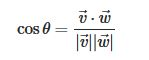# Torch.cross with a bigger dimension than 3?

Hi everybody,

Im trying to obtain the angle sin from two vectors, Im doing it by the formula

result1 / (result2 * result3)

where result1 is the norm of the cross multiplication from the two vectors, result 2 and 3 are the norm of each vector.

but when executing torch.cross between the two vectors its always out of range, except only if im multiplying a 3 dimension vectors, in my case I handle a 500 dimension vectors.

Any idea ?

Thanks.

The error thrown in your line of code is because you are trying to calculate the cross product of two 1-dimensional tensors in dim4.
However, even if you fix it by using `torch.cross(m1, m2, 0)`, you’ll get the following error:

``````RuntimeError: dimension 0 does not have size 3
``````

I’m not a mathematician, but as far as I know the cross product only exists in 3- and 7-dimensional Euclidean space. W. S. Massey wrote a paper about it.

Would the cos formula for the angle calculation work for you or are you somehow bound to the sin formula?

``````angle = torch.acos(torch.dot(m1, m2) / (m1.norm() * m2.norm()))
``````

Thanks, actually I could use the cos angle to find the sin.

cos = torch.dot(m1, m2) / (m1.norm() * m2.norm())
sin = torch.sqrt(1-(cos*cos))